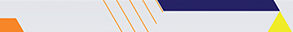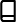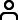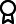Journal metrics
Acceptance rate15%
Submission to final decision42 days
Acceptance to publication64 days
CiteScore1.900
Journal Citation Indicator-
Impact Factor-

### Article of the Year 2020

Mathematical Modelling and Analysis of Transmission Dynamics of Lassa Fever

####Journal profile

Journal of Applied Mathematics publishes original research papers and review articles in all areas of applied, computational, and industrial mathematics.

####Editor spotlight

Chief Editor, Professor Theodore E. Simos, is based at Ulyanovsk State Technical University, Russia. His main research interest is the numerical analysis of differential equations.

####Special Issues

Do you think there is an emerging area of research that really needs to be highlighted? Or an existing research area that has been overlooked or would benefit from deeper investigation? Raise the profile of a research area by leading a Special Issue.

## Latest Articles

More articles
Research Article

### Stability Analysis of the Crank-Nicolson Finite Element Method for the Navier-Stokes Equations Driven by Slip Boundary Conditions

This paper is devoted to the study of numerical approximation for a class of two-dimensional Navier-Stokes equations with slip boundary conditions of friction type. The objective is to establish the well-posedness and stability of the numerical scheme in -norm and -norm for all positive time using the Crank-Nicholson scheme in time and the finite element approximation in space. The resulting variational structure dealing with is in the form of inequality, and obtaining -estimate is more involved because of the presence of the nondifferentiable term appearing at the boundary where slip occurs. We prove that the numerical scheme is stable in and -norms with the aid of different versions of discrete Grownwall lemmas, under a CFL-type condition. Finally, some numerical simulations are presented to illustrate our theoretical analysis.

Research Article

### Volatility Analysis of Exchange Rate with Correlated Errors: A Sliding Data Matrix Approach

The main objective of this study is to propose a method of analysing the volatility of a seemingly random walk time series with correlated errors without transforming the series as performed traditionally. The proposed method involves the computation of moving volatilities based on sliding and cumulative data matrices. Our method rests on the assumption that the number of subperiods for which the series is available is the same for all periods and on the assumption that the series observations in each subperiod for all the periods under consideration are a random sample from a particular distribution. The method was successfully implemented on a simulated dataset. A paired sample -test, Wilcoxon signed rank test, repeated measures (ANOVA), and Friedman tests were used to compare the volatilities of the traditional method and the proposed method under both sliding and cumulative data matrices. It was found that the differences among the average volatilities of the traditional method and sliding and cumulative matrix methods were insignificant for the simulated series that follow the random walk theorem. The implementation of the method on exchange rates for Canada, China, South Africa, and Switzerland resulted in adjudging South Africa to have the highest fluctuating exchange rates and hence the most unstable economy.

Research Article

### Positive Solutions for Third-Order Boundary Value Problems with the Integral Boundary Conditions and Dependence on the First-Order Derivatives

In this paper, by the use of a new fixed point theorem and the Green function of BVPs, the existence of at least one positive solution for the third-order boundary value problem with the integral boundary conditions is considered,where there is a nonnegative continuous function. Finally, an example which to illustrate the main conclusions of this paper is given.

Research Article

### Lagrange Multivariate Polynomial Interpolation: A Random Algorithmic Approach

The problems of polynomial interpolation with several variables present more difficulties than those of one-dimensional interpolation. The first problem is to study the regularity of the interpolation schemes. In fact, it is well-known that, in contrast to the univariate case, there is no universal space of polynomials which admits unique Lagrange interpolation for all point sets of a given cardinality, and so the interpolation space will depend on the set of interpolation points. Techniques of univariate Newton interpolating polynomials are extended to multivariate data points by different generalizations and practical algorithms. The Newton basis format, with divided-difference algorithm for coefficients, generalizes in a straightforward way when interpolating at nodes on a grid within certain schemes. In this work, we propose a random algorithm for computing several interpolating multivariate Lagrange polynomials, called RLMVPIA (Random Lagrange Multivariate Polynomial Interpolation Algorithm), for any finite interpolation set. We will use a Newton-type polynomials basis, and we will introduce a new concept called -partition. All the given algorithms are tested on examples. RLMVPIA is easy to implement and requires no storage.

Research Article

### Timelike -Surfaces in Minkowski 3-Space and the Sinh-Gordon Equation

Let and be two timelike surfaces in Minkowski 3-space . If there exists a spacelike (timelike) Darboux line congruence between each point of and , then the surfaces are timelike Weingarten surfaces. It turns out their Tschebyscheff angles are solutions of the Sinh-Gordon equation, and the surfaces are related to each other by Backlund’s transformation. Finally, a method to construct new timelike Weingarten surface has been found.

Research Article

### Optimal Control Model for Alcohol–Related Risk Behaviors and Beliefs in Tanzania

An optimal control theory is applied to a system of ordinary differential equations representing the dynamics of health-related risks associated with alcoholism in the community with active religious beliefs. Two nonautonomous control variables are proposed to reduce the health risks associated with alcoholism in the community. Consequently, three control strategies are presented using Pontryagin’s maximum principle (PMP), and the necessary conditions for the existence of the optimal controls are obtained. The simulation results revealed that the health risks associated with alcoholism behaviors may be effectively eradicated when both controls, and , are applied in a combination. On the other hand, the cost–effective analysis of the three different strategies confirmed that the desired cost–effective results may be attained when both controls, and , are applied together. Based on these results, this study concludes that, health risks associated with alcoholism behaviors may be efficiently and cost–effectively eradicated from the community when both controls, and , are applied together. Whereas application of control option implies increasing the level of protection to the susceptible population by implementing public health education campaign; the control option implies increasing the removal rate of the moderate risky individuals into recovered population. The control strategy in which the two options are featured in a combination is presented in this study as Strategy C exhibiting the least ICER value and more cost–effective than the rest of strategies presented.Journal metrics
Acceptance rate15%
Submission to final decision42 days
Acceptance to publication64 days
CiteScore1.900
Journal Citation Indicator-
Impact Factor-Author guidelinesEditorial boardDatabases and indexingAuthor guidelinesEditorial boardDatabases and indexing

Article of the Year Award: Outstanding research contributions of 2020, as selected by our Chief Editors. Read the winning articles.